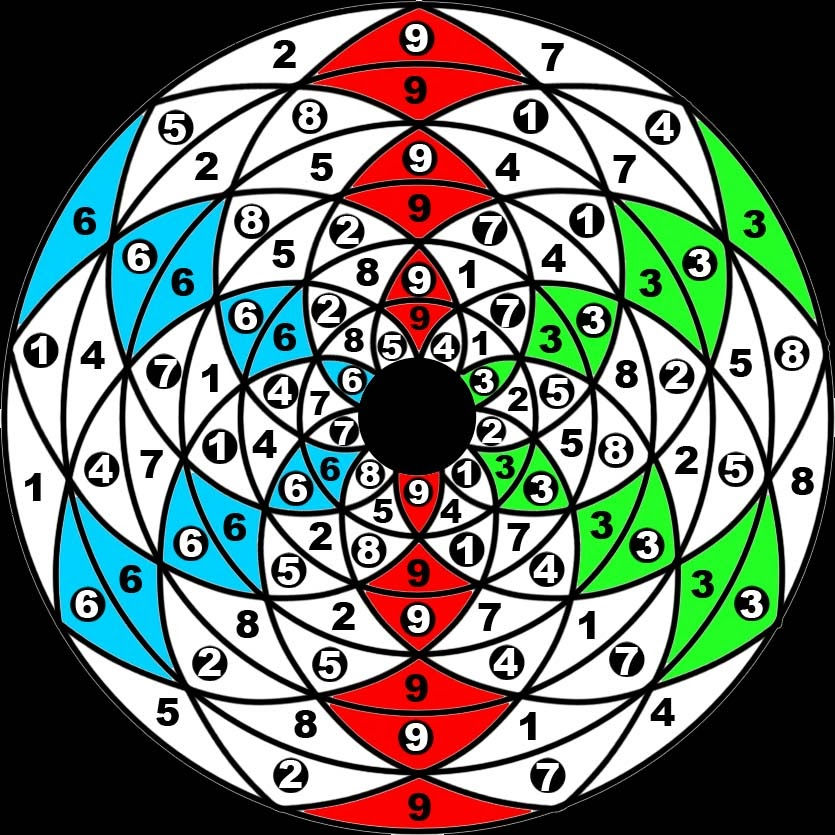top of page
Search

# Enneagram star tetrahedron tree of life superstring theory

Tetragrammaton/tetractys/10dimensions/star tetrahedron/tree of life/seed of life/fingerprint of god/enneagram/triangle numbers:124875=142857=27

124875+142857=267732

267732=2:77:2=(77:77):2=28:2=28:28=28

(64 tetrahedron grid(2tetragrammatons))=28

(8 tree of life's)=(64 tetrahedron grid)=(metatrons cube)=(fruit of life)